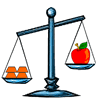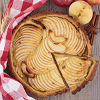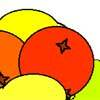#### You may also like### What's My Weight?

There are four equal weights on one side of the scale and an apple on the other side. What can you say that is true about the apple and the weights from the picture?### Pies

Grandma found her pie balanced on the scale with two weights and a quarter of a pie. So how heavy was each pie?### Oranges and Lemons

On the table there is a pile of oranges and lemons that weighs exactly one kilogram. Using the information, can you work out how many lemons there are?

# Money Measure

##### Age 7 to 11Challenge Level

Excellent solutions were received from several of you including Heather from Stow Heath Junior School, Konrad from Baulkham Hills High and Richard from Sullivan School. Heather explains how you might go about finding the odd box:

Take one coin from the first box, two coins from the second box and so on. You will end up with 55 coins taken from the various boxes. Weigh the 55 coins. If the weight reads one gram less than 550 then 1 coin weighs 9 grams and box number 1 contains the light coins. If the weight reads 2 grams less than 550 then two coins weigh 9 grams and box number two contains the light coins and so on.

Konrad puts it a slightly different way:

The last digit of the weight will tell you which box has the 9g coins in it. If the last digit is 9 then the box you took 1 coin out of is the box you are looking for. If the last digit is 8 then the box you took 2 coins out of is the box you are looking for. If the last digit is 7 then the box you took 3 coins out of is the box you are looking for and so on.

Thank you to everyone for your solutions and sorry that we can't mention you all.